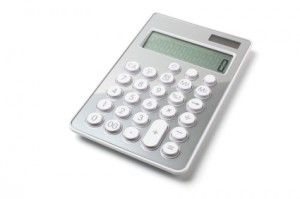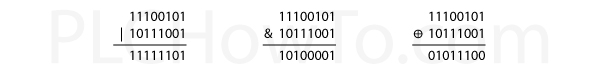# PLC MathThis page assumes you understand the principles discussed on the Data Inside PLCs page.

### The Basics

Addition, Subtraction, Multiplication and Division are what you’d expect.  If you haven’t done math in a programming language before, there are a couple of things you should be aware of.

• Overflow:  Integers and Floating Point Numbers have size limits.  When you go beyond their size you’ll cause yourself problems.
Example: 8 bit Unsigned Int with value 255 in bits looks like 11111111. If we add 1 to it we get 100000000. There isn’t storage for a 9’th bit, so we’re left with 00000000 or 0. So in this case 255 + 1 = 0
Another Example: 8 bit Unsigned Int with value 0 in bits looks like 00000000. If we subtract 1 to it we get 11111111. So, we can get the opposite problem 0 – 1 = 255
• Rounding:  Floating Point numbers aren’t perfect.  For this reason, you shouldn’t check to see if Floating point numbers are equal to a value, you should check to see if it’s within a range of numbers.
Example: 2 * 3 performed with Floating Point numbers may not result in exactly 6.
It could be 5.9999999999998 or something similar. So instead of asking is Example = 6, ask is Example greater than 5.9 and less than 6.1. Don’t worry about how to ask these questions, we’ll talk about that later in the tutorial

### Boolean Math

Some programming packages allow bit manipulation by using Boolean Math.  The main operations are:

OR (symbol ‘|’ ):  A = B | C.  If either B is 1 or C is 1, then A is 1.  If both B and C are 0, then A is 0.

AND (symbol ‘&’): A = B & C.  If both B and C are 1, then A is 1.  If either B of C are 0, then A is 0.

Exclusive OR (symbol ‘⊕’):  A = B ⊕ C.  If either B is 1 or C is 1, but not both, then A is 1.  If both B and C are 1, or both B and C are 0 then A is 0.

Boolean Math can be performed on Integers.
Examples using two Unsigned 8-bit Integers 229 (11100101 in binary), and 185 (10111001 in binary):